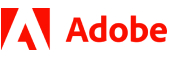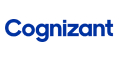New update is available. Click here to update.

# Squares of a Sorted Array

Easy0/40
15 mins
85 %42 upvotes+8 more

## Problem Statement

#### For example :

``````Input:
‘ARR’ = [-6,-3, 2, 1, 5]

If we take a square of each element then the array/list will become [36, 9, 4, 1, 25].
Then the sorted array/list will be [1, 4, 9, 25, 36].

Output :
[1, 4, 9, 25, 36].
``````
Detailed explanation ( Input/output format, Notes, Images )

#### Sample Input 1:

``````2
4
-3 -3 1 2
6
0 1 2 3 4 5
``````

#### Sample Output 1:

``````1 4 9 9
0 1 4 9 16 25
``````

#### Explanation Of Sample Input 1:

``````For test case 1:
On taking the square of each element ‘ARR’ will become [9, 9, 1, 4].
Now we can sort the array/list and output will be [1, 4, 9, 9].

For test case 2:
On taking the square of each element ‘ARR’ will become [0, 1, 4, 9, 16, 25].
Now we can see that the array/list is already sorted, so the output will be  [0, 1, 4, 9, 16, 25].
``````

#### Sample Input 2:

``````2
1
5
4
-6 -3 -2 -1
``````

#### Sample Output 2:

``````25
1 4 9 36
``````

#### Explanation Of Sample Input 2:

``````For test case 1:
On taking the square of each element ‘ARR’ will become .
Now as the array/list has only 1 element so it is already sorted, the output will be .

For test case 2:
On taking the square of each element ‘ARR’ will become [36,9,4,1].
Now we can see that the array/list is already sorted, so the output will be [36, 9, 4, 1].
``````AutocompleteConsole